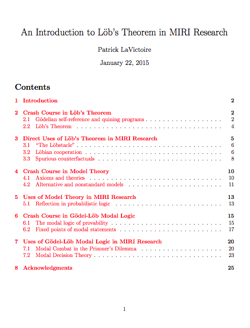# New report: “An Introduction to Löb’s Theorem in MIRI Research”

|   |  PapersToday we publicly release a new technical report by Patrick LaVictoire, titled “An Introduction to Löb’s Theorem in MIRI Research.” The report’s introduction begins:

This expository note is devoted to answering the following question: why do many MIRI research papers cite a 1955 theorem of Martin Löb, and indeed, why does MIRI focus so heavily on mathematical logic? The short answer is that this theorem illustrates the basic kind of self-reference involved when an algorithm considers its own output as part of the universe, and it is thus germane to many kinds of research involving self-modifying agents, especially when formal verification is involved or when we want to cleanly prove things in model problems. For a longer answer, well, welcome!

I’ll assume you have some background doing mathematical proofs and writing computer programs, but I won’t assume any background in mathematical logic beyond knowing the usual logical operators, nor that you’ve even heard of Löb’s Theorem before.

If you’d like to discuss the article, please do so here.